### Light – Reflection And Refraction Class 10th Science CBSE Solution

##### Question 1.Which one of the following materials cannot be used to make a lens?A. waterB. glassC. plasticD. clayAnswer:Clay cannot be used to make a lens. Because it is opaque material, and light cannot pass through it.Question 2.The image formed by a concave mirror is observed to be virtual, erect and larger than the object. Where should be the position of the object?A. between the principal focus and the centre of curvatureB. at the centre of curvatureC. beyond the centre of curvatureD. between the pole of the mirror and its principal focus.Answer:When the image formed by a concave mirror is observed to be virtual, erect and larger than the object, the position of image will be between the pole of the mirror and its principal focusQuestion 3.Where should an object be placed in front of a convex lens to get a real image of the size of the object ?A. at the principal focus of the lensB. at twice the focal lengthC. at infinityD. between the optical center of the lens and its principal focusAnswer:The object should be placed at twice the focal length to get a real image of the size of the object. Thus, option (b) is correct.Question 4.A spherical mirror and a thin spherical lens each have a focal length of, -15 cm. The mirror and the lens are likely to be:A. both concaveB. both convexC. the mirror is concave and the lens is convexD. the mirror is convex but the lens is concaveAnswer:A spherical mirror and a thin spherical lens each having a focal length of -15 cm are both concave.Question 5.No matter how far you stand from a mirror, your image appears erect. The mirror is likely to be:A. planeB. concaveC. convexD. either plane or convexAnswer:Plane mirror or convex mirror both form erect images. Thus, option (d) is correct.Question 6.Which of the following lenses would you prefer to use while reading small letters found in a dictionary?A. A convex lens of focal length 50 cmB. A concave lens of focal length 50 cmC. A convex lens of focal length 5 cmD. A concave lens of focal length 5 cmAnswer:A convex lens of focal length 5 cm is used for reading small letters. Thus, option (c) is correct.Question 7.We wish to obtain an erect image of an object using a concave mirror of focal length 15 cm. What should be the range of distance of the object from the mirror? What is the nature of the image? Is the image larger or smaller than the object? Draw a ray diagram to show the image formation in this case.Answer:In order to obtain an erect image of an object with a concave mirror, the object should be at a distance less than its focal length as seen below: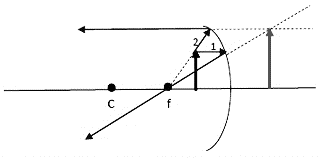Here the focal length of concave mirror is 15 cm. So to obtain an erect image the object by using this concave mirror, the object should be placed at any distance which is less than 15 cm from the mirror. The nature of image will be virtual. The image will be larger than the object.Question 8.Name the type of mirror used in the following situations :(a) Headlights of a car(b) Side/rear view mirror of a vehicle(c) Solar furnaceSupport your answer with reason.Answer:(a) A concave mirror is used in the headlights of a car. This is because when, a lit bulb is placed at the focus of the concave reflector, then the concave reflector produces a powerful beam of parallel light rays. This beam of light helps us to see things up to a considerable distance in the darkness of night.(b) A convex mirror is used as side-view mirror or rear-view mirror in a vehicle. This is because:(i) A convex mirror always produces an erect image of the objects(ii) the image formed in a convex mirror is highly diminished due to which a convex mirror gives a wide field of view of the traffic.(c) A concave mirror is used in a solar furnace. This is because when the solar furnace is placed at the focus of a large concave reflector, then the concave reflector converges and focuses the sun's heat rays on the furnace due to which the solar furnace gets very hot.Question 9.One half of a convex lens is covered with a black paper. Will this lens produce a complete image of the object ? Verify your answer experimentally. Explain your observations.Answer:When a convex lens is half covered with a black paper. It will produce a complete image of an object because light rays can still pass through its optical centre (as shown in figure given here).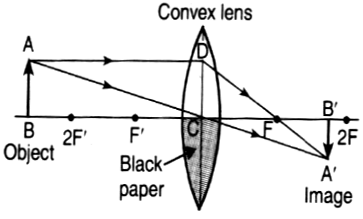Question 10.An object 5 cm in length is held 25 cm away from a converging lens of focal length 10 cm. Draw the ray diagram and find the position, size and nature of the image formed.Answer:A converging lens means a convex lens. The diagram is shown below: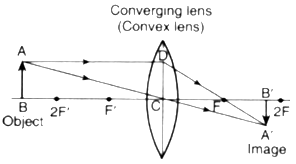Here, Object distance, u = - 25 cmImage distance, v = ? (To be calculated)And, Focal length, f = + 10 cm (because it is convex lens)Now, putting these values in the lens formula: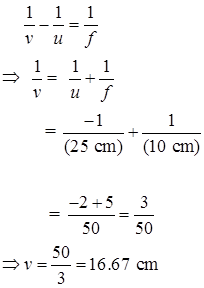Thus, the position of image is at a distance of 16.67 cm from the lens. The plus sign for image distance shows that the image is formed on the right side of lens (or behind the lens) and that the nature of image is real and inverted.Let us calculate the magnification now. For a lens:Magnification,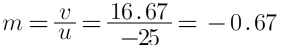We will now calculate the size of image h2 by knowing the size of object h1 and value of m:Now,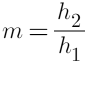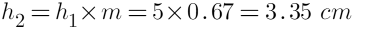Thus, the size of image is 3.35 cm. The negative sign of the magnification shows that the image is inverted.Question 11.A concave lens of focal length 15 cm forms an image 10 cm from the lens. How far is the object from the lens ? Draw the ray diagram.Answer:Here, Object distance, u = ? (To be calculated)Image distance, v =- 10 cm (To the left of concave lens)Focal length, f =- 15 cm (It is a concave lens)Now, putting these values in the lens formula: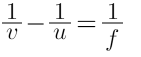Putting the values of v and f in the above equation, we get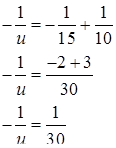Object distance, u = -30 cmThus, the object is placed at a distance of 30 cm from the concave lens. The minus sign with object distance shows that the object is on its left side. The diagram is shown as: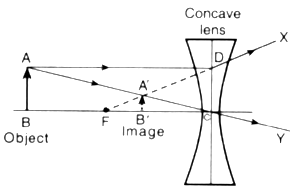Question 12.An object is placed at a distance of 10 cm from a convex mirror of focal length 15 cm. Find the position and nature of the image.Answer:We can find the position of image by calculating the image distance, v.Here, Object distance, u =- 10 cm (To the left of mirror)Image distance, v = ? (To be calculated)And, Focal length, f = +15 cm (It is a convex mirror)Putting these values in the mirror formula: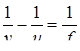We get: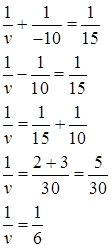So, Image distance, v = + 6cmThus, the position of image is at a distance of 6 cm from the convex mirror on its right side (behind the mirror). Since the image is formed behind the convex mirror, therefore, the nature of image is virtual and erect.Question 13.The magnification produced by a plane minor is +L What does this mean ?Answer:The plus sign (+) of the magnification shows that the image is virtual and erect. And the value 1 for magnification shows that the image is exactly of the same size as the object. So, the magnification of +1 produced by a plane mirror means that the image formed in a plane mirror is virtual and erect, and of the same size as the object.Question 14.An object 5.0 cm in length is placed at a distance of 20 cm in front of a convex mirror of radius of curvature 30 cm. Find the position of the image, its nature and size.Answer:Here, Object distance, u = -20 cm (to the left of mirror)Image distance, v = ? (To be calculated)Radius of curvature, R = +30cm (It is a convex mirror)So, Focal length,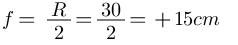Now, putting these values of u and applying formula: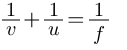We get: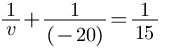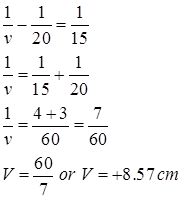Thus, the position of image is 8.57 cm behind the mirror (on its right side). Since the image is formed behind the convex mirror, therefore, the nature of image is virtual and erect.Now,For a mirror, Magnification, m =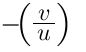So, m =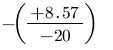= + 0.42Also, Magnification, m =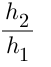Where h2= Height of image, h1= Height of ObjectSo, +0.42 =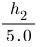h2 = 0.42×5.0 = 2.1 cmThus, the size of image is 2.1 cm.Question 15.An object of size 7.0 cm is placed at 27 cm in front of a concave mirror of focal length 18 cm. At what distance from the mirror should a screen be placed so that a sharp focused image can be obtained? Find the size and nature of the image.Answer:Here, Object distance, u = -27 cm (To the left side of mirror)Image distance, v = ? (To be calculated)Focal length, f = -18 cm (It is a concave mirror)Height of object, h1 = 7.0 cmAnd, Height of image, h2 = ? (To be calculated)Now, For a mirror: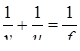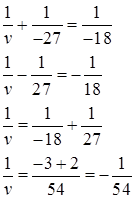Image distance, v = -54 cmSince the image distance is minus 54 cm, therefore, the screen should be placed at a distance of 54 cm in front of the concave mirror (on its left side). The nature of image obtained on the screen is real and inverted.Also, For a mirror,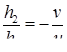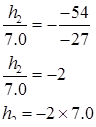= -14.0 cmThus, the size of image is 14.0 cm.Question 16.Find the focal length of a lens of power, -2.0 D. What type of lens is this?Answer:The power of this lens has a negative sign (or minus sign), indicating its a concave lens. Now,Power, P =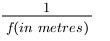(f= Focal Length)So, -2.0 =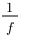And,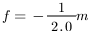Or,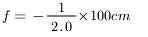So, Focal length of lens, f = -50 cmQuestion 17.A doctor has prescribed a corrective lens of power + 1.5 D. Find the focal length of the lens. Is the prescribed lens diverging or converging?Answer:The power of this lens is positive (with a plus sign), therefore, this is a convex lens or converging lens. Now,Power, P =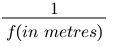So, +1.5 =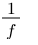∴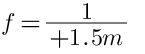⇒ f=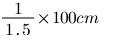So, Focal length of lens, f = +66.7 cm.

PDF FILE TO YOUR EMAIL IMMEDIATELY PURCHASE NOTES & PAPER SOLUTION. @ Rs. 50/- each (GST extra)

HINDI ENTIRE PAPER SOLUTION

MARATHI PAPER SOLUTION

SSC MATHS I PAPER SOLUTION

SSC MATHS II PAPER SOLUTION

SSC SCIENCE I PAPER SOLUTION

SSC SCIENCE II PAPER SOLUTION

SSC ENGLISH PAPER SOLUTION

SSC & HSC ENGLISH WRITING SKILL

HSC ACCOUNTS NOTES

HSC OCM NOTES

HSC ECONOMICS NOTES

HSC SECRETARIAL PRACTICE NOTES

# 2019 Board Paper Solution

HSC ENGLISH SET A 2019 21st February, 2019

HSC ENGLISH SET B 2019 21st February, 2019

HSC ENGLISH SET C 2019 21st February, 2019

HSC ENGLISH SET D 2019 21st February, 2019

SECRETARIAL PRACTICE (S.P) 2019 25th February, 2019

HSC XII PHYSICS 2019 25th February, 2019

CHEMISTRY XII HSC SOLUTION 27th, February, 2019

OCM PAPER SOLUTION 2019 27th, February, 2019

HSC MATHS PAPER SOLUTION COMMERCE, 2nd March, 2019

HSC MATHS PAPER SOLUTION SCIENCE 2nd, March, 2019

SSC ENGLISH STD 10 5TH MARCH, 2019.

HSC XII ACCOUNTS 2019 6th March, 2019

HSC XII BIOLOGY 2019 6TH March, 2019

HSC XII ECONOMICS 9Th March 2019

SSC Maths I March 2019 Solution 10th Standard11th, March, 2019

SSC MATHS II MARCH 2019 SOLUTION 10TH STD.13th March, 2019

SSC SCIENCE I MARCH 2019 SOLUTION 10TH STD. 15th March, 2019.

SSC SCIENCE II MARCH 2019 SOLUTION 10TH STD. 18th March, 2019.

SSC SOCIAL SCIENCE I MARCH 2019 SOLUTION20th March, 2019

SSC SOCIAL SCIENCE II MARCH 2019 SOLUTION, 22nd March, 2019

XII CBSE - BOARD - MARCH - 2019 ENGLISH - QP + SOLUTIONS, 2nd March, 2019

# HSCMaharashtraBoardPapers2020

(Std 12th English Medium)

HSC ECONOMICS MARCH 2020

HSC OCM MARCH 2020

HSC ACCOUNTS MARCH 2020

HSC S.P. MARCH 2020

HSC ENGLISH MARCH 2020

HSC HINDI MARCH 2020

HSC MARATHI MARCH 2020

HSC MATHS MARCH 2020

# SSCMaharashtraBoardPapers2020

(Std 10th English Medium)

English MARCH 2020

HindI MARCH 2020

Hindi (Composite) MARCH 2020

Marathi MARCH 2020

Mathematics (Paper 1) MARCH 2020

Mathematics (Paper 2) MARCH 2020

Sanskrit MARCH 2020

Sanskrit (Composite) MARCH 2020

Science (Paper 1) MARCH 2020

Science (Paper 2)

Geography Model Set 1 2020-2021

MUST REMEMBER THINGS on the day of Exam

Are you prepared? for English Grammar in Board Exam.

Paper Presentation In Board Exam

How to Score Good Marks in SSC Board Exams

Tips To Score More Than 90% Marks In 12th Board Exam

How to write English exams?

How to prepare for board exam when less time is left

How to memorise what you learn for board exam

No. 1 Simple Hack, you can try out, in preparing for Board Exam

How to Study for CBSE Class 10 Board Exams Subject Wise Tips?

JEE Main 2020 Registration Process – Exam Pattern & Important Dates

NEET UG 2020 Registration Process Exam Pattern & Important Dates

How can One Prepare for two Competitive Exams at the same time?

8 Proven Tips to Handle Anxiety before Exams!